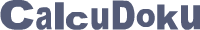﻿ CalcuDoku
 Log inBecome a member# CalcuDoku

## Addition, subtraction, multiplication and division will never be the same again

• The math teaching puzzle from Japan
• Fun, addictive and fascinating
• Simple rules, very easy to learn
• Requires no language skills
• Wide range of logic and difficulty levels
• Develops logical deduction and reasoning

# SingleOp CalcuDoku

Play new puzzles each week

Each puzzle consists of a grid containing blocks surrounded by bold lines. The object is to fill all empty squares so that the numbers 1 to N (where N is the number of rows or columns in the grid) appear exactly once in each row and column and the numbers in each block produce the result shown in the top-left corner of the block according to the math operation appearing on the top of the grid. In CalcuDoku a number may be used more than once in the same block.

SingleOp CalcuDoku 4x4.a.n
Difficulty: Ultra easy
SingleOp CalcuDoku 5x5.m.n
Difficulty: Very easy
SingleOp CalcuDoku 6x6.a.n
Difficulty: Medium

# DualOp CalcuDoku

Play new puzzles each week

Each puzzle consists of a grid containing blocks surrounded by bold lines. The object is to fill all empty squares so that the numbers 1 to N (where N is the number of rows or columns in the grid) appear exactly once in each row and column and the numbers in each block produce the result of the math operation shown in the top-left corner of the block. In CalcuDoku a number may be used more than once in the same block.

DualOp CalcuDoku 4x4.as.a
Difficulty: Ultra easy
DualOp CalcuDoku 5x5.as.a
Difficulty: Easy
DualOp CalcuDoku 6x6.md.a
Difficulty: Medium